Function Repository Resource:

# CurveIntersection

Find the points of intersection of two curves

Contributed by: Wolfram|Alpha Math Team
 ResourceFunction["CurveIntersection"][{expr1,expr2},{x,y}] returns the intersections of implicit curves expr1 and expr2 as points in the xy-plane. ResourceFunction["CurveIntersection"][{expr1,expr2},{x,y},dom] returns the intersections of expr1 and expr2 as points in the xy-plane, considered over the domain dom. ResourceFunction["CurveIntersection"][{expr1,expr2},{x,y},dom,type] returns the intersections with format type.

## Details and Options

The domain dom may be Reals, Complexes or Integers and defaults to Complexes.
The argument type may be Grid, List, Values or "Indexed" and defaults to List.
ResourceFunction["CurveIntersection"] accepts the option "Constraints" which can specify constraints on the input variables.

## Examples

### Basic Examples (2)

Obtain the points of intersection of x+1 and 2x2:

 In:=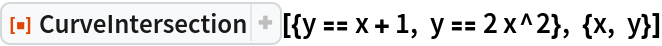Out=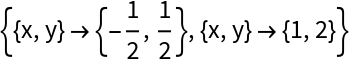Find the intersections of implicitly defined curves:

 In:=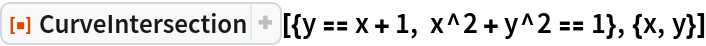Out=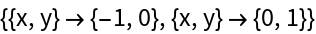### Scope (3)

Find the integer-valued intersections of two implicitly defined curves:

 In:=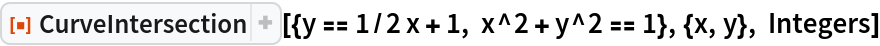Out=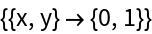If there is no intersection, CurveIntersection returns unevaluated:

 In:=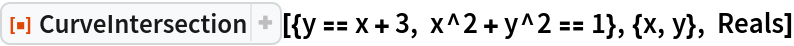Out=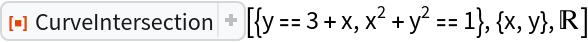The fourth argument changes the format of the output:

 In:=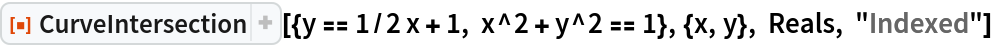Out=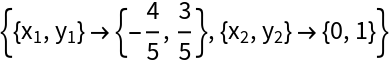### Options (1)

Find the intersections of two curves given some constraints:

 In:=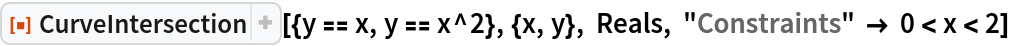Out=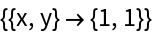In:=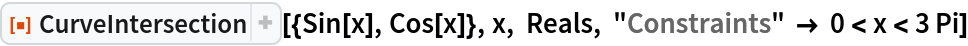Out=### Possible Issues (1)

For curves that intersect infinitely many times, a subset of the points of intersection will be returned:

 In:=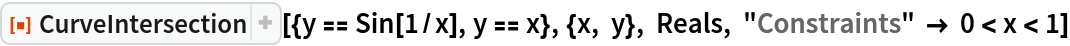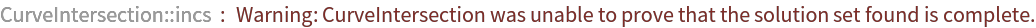Out=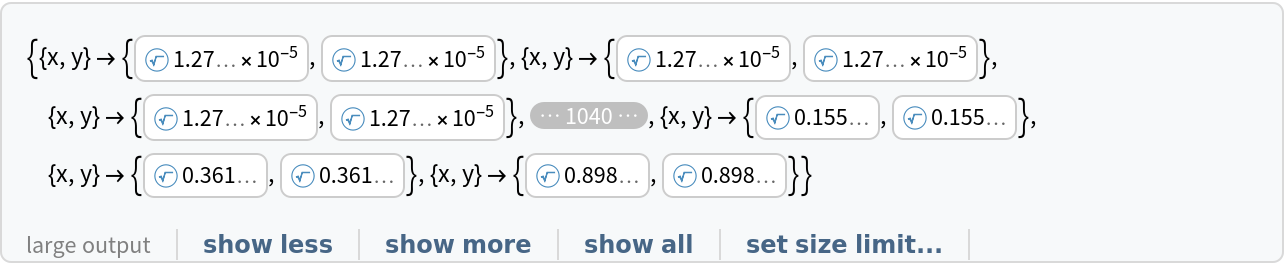## Publisher

Wolfram|Alpha Math Team

## Version History

• 2.0.0 – 23 March 2023
• 1.0.0 – 01 April 2020